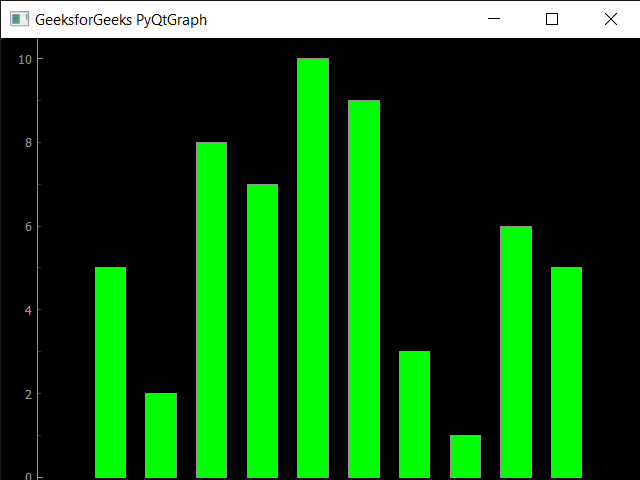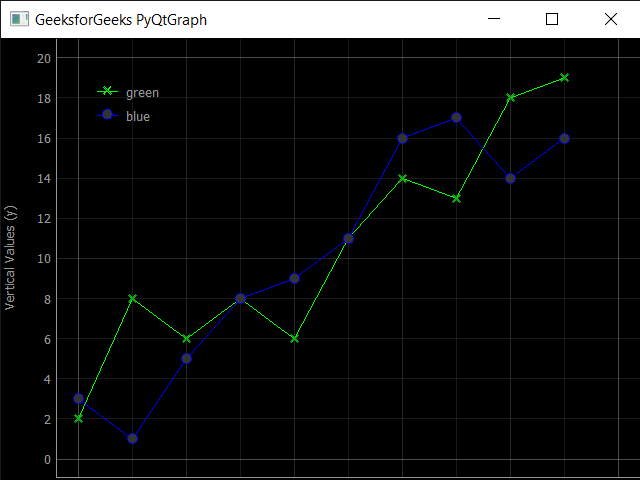Related Articles
Introduction to PyQtGraph Module in Python
• Difficulty Level : Medium
• Last Updated : 14 Oct, 2020

PyQtGraph is a graphics and user interface library for Python that provides functionality commonly required in designing and science applications. Its primary goals are to provide fast, interactive graphics for displaying data (plots, video, etc.) and second is to provide tools to aid in rapid application development (for example, property trees such as used in Qt Designer).

PyQtGraph makes heavy use of the Qt GUI platform (via PyQt or PySide) for its high-performance graphics and numpy for heavy number crunching. In particular, pyqtgraph uses Qt’s GraphicsView framework which is a highly capable graphics system on its own, this brings optimized and simplified primitives to this framework to allow data visualization with minimal effort.

In order to install the PyQtGraph we use the command given below

```pip install pyqtgraph
```

Requirements: PyQtGraph can run on any platform which supports the following packages:
1. Python 2.7 and 3+
2. PyQt 4.8+ or PySide
3. NumPy
4. python-opengl bindings are required for 3D graphics

Example : In this example we will create a simple bar graph using PyQtGraph module in python

 `# importing pyqtgraph as pg``import` `pyqtgraph as pg`` ` `# importing QtCore and QtGui from the pyqtgraph module``from` `pyqtgraph.Qt ``import` `QtCore, QtGui`` ` `# importing numpy as np``import` `numpy as np`` ` `# creating a pyqtgraph plot window``window ``=` `pg.plot()`` ` `# title``title ``=` `"GeeksforGeeks PyQtGraph"`` ` `# setting window title``window.setWindowTitle(title)`` ` `# create list for y-axis``y1 ``=` `[``5``, ``5``, ``7``, ``10``, ``3``, ``8``, ``9``, ``1``, ``6``, ``2``]`` ` `# create horizontal list i.e x-axis``x ``=` `[``1``, ``10``, ``4``, ``5``, ``7``, ``3``, ``6``, ``8``, ``9``, ``2``]`` ` `# create pyqt5graph bar graph item ``# with bar colors = green``bargraph1 ``=` `pg.BarGraphItem(x ``=` `x, height ``=` `y1, width ``=` `0.6``, brush ``=``'g'``)`` ` `# adding bargraph item to the window``window.addItem(bargraph1)`` ` `# main method``if` `__name__ ``=``=` `'__main__'``:``     ` `    ``# importing system``    ``import` `sys``     ` `    ``# Start Qt event loop unless running in interactive mode or using ``    ``if` `(sys.flags.interactive !``=` `1``) ``or` `not` `hasattr``(QtCore, ``'PYQT_VERSION'``):``        ``QtGui.QApplication.instance().exec_()`

Output :Example : In this we will plot two lines together in same graph, below is the implementation

 `# importing pyqtgraph as pg``import` `pyqtgraph as pg`` ` `# importing QtCore and QtGui from the pyqtgraph module``from` `pyqtgraph.Qt ``import` `QtCore, QtGui`` ` `# importing numpy as np``import` `numpy as np`` ` `# define the data``title ``=` `"GeeksforGeeks PyQtGraph"`` ` `# y values to plot by line 1``y ``=` `[``2``, ``8``, ``6``, ``8``, ``6``, ``11``, ``14``, ``13``, ``18``, ``19``]`` ` `# y values to plot by line 2``y2 ``=` `[``3``, ``1``, ``5``, ``8``, ``9``, ``11``, ``16``, ``17``, ``14``, ``16``]``x ``=` `range``(``0``, ``10``)`` ` `# create plot object``plt ``=` `pg.plot()`` ` `# showing x and y grids``plt.showGrid(x ``=` `True``, y ``=` `True``)`` ` `# adding legend``plt.addLegend()`` ` `# set properties of the label for y axis``plt.setLabel(``'left'``, ``'Vertical Values'``, units ``=``'y'``)`` ` `# set properties of the label for x axis``plt.setLabel(``'bottom'``, ``'Horizontal Vlaues'``, units ``=``'s'``)`` ` `# setting horizontal range``plt.setXRange(``0``, ``10``)`` ` `# setting vertical range``plt.setYRange(``0``, ``20``)`` ` `# setting window title``plt.setWindowTitle(title)`` ` `# ploting line in green color``line1 ``=` `plt.plot(x, y, pen ``=``'g'``, symbol ``=``'x'``, symbolPen ``=``'g'``, symbolBrush ``=` `0.2``, name ``=``'green'``)`` ` `# ploting line2 with blue color``line2 ``=` `plt.plot(x, y2, pen ``=``'b'``, symbol ``=``'o'``, symbolPen ``=``'b'``, symbolBrush ``=` `0.2``, name ``=``'blue'``)`` ` ` ` ` ` `# main method``if` `__name__ ``=``=` `'__main__'``:``     ` `    ``# importing system``    ``import` `sys``     ` `    ``# Start Qt event loop unless running in interactive mode or using ``    ``if` `(sys.flags.interactive !``=` `1``) ``or` `not` `hasattr``(QtCore, ``'PYQT_VERSION'``):``        ``QtGui.QApplication.instance().exec_()`

Output :Things PyQtGraph can perform :
1. Basic data visualization like images, line and scatter plots
2. Fast enough to plot realtime update of video / data
3. Interactive scaling/panning, averaging, FFTs, SVG/PNG export
4. Widgets for marking & selecting plot regions
5. Automatic slicing of multi-dimensional image data
6. Framework for building customized image region-of-interest widgets
7. Docking system that replaces/complements Qt’s dock system to allow more complex (and more predictable) docking arrangements
8. ParameterTree widget for rapid prototyping of dynamic interfaces

Attention geek! Strengthen your foundations with the Python Programming Foundation Course and learn the basics.

To begin with, your interview preparations Enhance your Data Structures concepts with the Python DS Course. And to begin with your Machine Learning Journey, join the Machine Learning – Basic Level Course

My Personal Notes arrow_drop_up